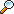Frothingham Electronics Corp Customer SupportTests:

# FEC200 PCV (Predict Clamping Voltage)Test

This test for FEC200 testers is intended to reject those TVS diodes that would likely fail the 10X1000 Clamping Voltage test. It is not intended to guarantee that any part will pass this test.

A TYPICAL TEST:

• TEST 5 PCV 10MA 100A <15V T1500 K900

10MA is the current at which the cold VZ is measured. 100A is the peak current that would be used in the real 10X1000 test. T1500 sets the pulse width used by PCV at the highest test current to 1500µS. K900 is a multiplier that you set for each type of part to fine tune the correlation of this test with the real VCC test. If you don't enter a K factor the default of 1000 will be used. 1000 represents a multiplier of 1.000.

### HOW IT WORKS

PCV predicts clamping voltage by extrapolating from a series of reading taken at much lower current levels than the real test.

Parts that fail the real 10X1000 test do so because the voltage rises above VZ that is due to both resistive and thermal components. The PCV test uses a moderately high test current that causes significant heating. It measures the delta VZ due to this heating as well as that due to the resistive component of the VZ/IZ characteristic.

First the VZ is measured at a low current such as the 10mA in the example. This value should be high enough to be over the knee of the TVS but not high enough to fause serious heading with short pulse widths.

Next the current is increased to 10% of the current calculated and a new VZ reading is taken and remembered. Finally the current increased to the calculated value and after the specified pulse width the VZ is measured again.

The delta voltage between these two readings is then multiplied by the ratio of the specified current for the real test and the current actually used. The result is then multiplied by a factor that is a constant, which can be set globally by the user. This constant is named PCVMULT and can be set in the file CUSTOM.TXT. Finally the reading is multiplied by the K factor if you entered one.

If the part tested was perfectly linear, no multiplier would be needed. We know however that parts are not linear. If no multiplier was used the predicted VCC would be far too high. The scale of the PVCMULT is such that 1000 is equivalent to 1.000.

In order to get the best possible correlation between PCV and the 10X1000 test, you should evaluate the combined effects of PCVMULT and the programmed pulse width.

The rise of voltage due to resistive slope would be independent of pulse width if it were not for the temperature increase and the TC of VZ. Therefore a very short pulse would measure primarily the resistive slope and a long pulse would be substantially affected by the Thermal Response of the part. By programming the pulse width (range 0.5-10mS) you can give this thermal effect its appropriate weight.

Frothingham Electronics Corporation
44 Crystal Lane
PO Box 629
Wakefield, NH 03872-0629
FAX: (603) 522-9710
Phone: (603) 522-6620

email Frothingham
email Webmaster
Sitemap.html
Last Updated September 12, 2007